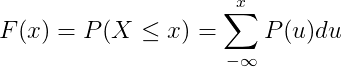# Probability Distribution

In probability theory and statistics, a probability distribution is a mathematical function that provides the probabilities of occurrence of different possible outcomes in an experiment. In more technical terms, the probability distribution is a description of a random phenomenon in terms of the probabilities of events.

Contents

### Cumulative distribution function

Because a probability distribution P on the real line is determined by the probability of a scalar random variable X being in a half-open interval (−∞, x], the probability distribution is completely characterized by its cumulative distribution function:

F(x) = P(X ≤ x)

for all R

Continuous distribution

The cumulative distribution function F(x) is calculated by integration of the probability density function f(u) of continuous random variable X.Discrete distribution

The cumulative distribution function F(x) is calculated by summation of the probability mass function P(u) of discrete random variable X.